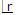Click to Chat

1800-1023-196

+91-120-4616500

CART 0

• 0

MY CART (5)

Use Coupon: CART20 and get 20% off on all online Study Material

ITEM
DETAILS
MRP
DISCOUNT
FINAL PRICE
Total Price: Rs.

There are no items in this cart.
Continue Shopping```Useful Tips for Algebra

Some Useful Tips(i) Number of combinations of dissimilar things taken r at a time when p particular things always occur = n-pCr-p.

Explanation: Here, actually we are making a selection of (r - p) things out of (n - p) things which can be done in n-pCr-p ways.

(ii) Number of permutations of n dissimilar things taken r at a time when p particular things always occur = n-pCr-pr!

Explanation: Here, number of combinations is the same as above but every combinations of r things can be permutated inways and that's why total number of permutations = n-pCr-pr!

(iii) Number of combinations of n dissimilar things taken r at a time when p particular things never occur = n-pCr-p.

Explanation: Here, actual selection of r things is being made out of (n - p) things and that's why total number of selections = n-pCr-p.

(iv) Number of permutations of n dissimilar things taken r at a time when p particular things never occur = n-pCr-p.

Explanation: Here, number of combinations is the same as above but every selection made of r things can be permutated inays and therefore then total no. of permutations = n-pCr-p.

(v) Gap Method: If there are m men and n women (m > n) and they have to sit in a row in such a way that no two women sit together then total no. of such arrangements = m+1Cn. m!

Explanation: If we denote men by m and women by w then there are exactly (m + 1) places in which women can be placed such that no two women will be together. This can be done in m+1Cn ways. Moreover, m men can be arranged among themselves in m! ways. Therefore, total number of arrangements : m+1Cn.m!

w m w m w .................. m w m w

(vi)   String method: Many a times, one may encounter a problem of arranging n number of persons in a row such that m of them is always together. For this we tie all these m persons with a string i.e. we treat them as one, then we have (n - m + 1). Therefore total number of arrangements = (n - m + 1)!.m!.

Never together: If question is to find the number of arrangements such that m out of n are never all together then such total number of arrangements.

Total possible arrangements of a n persons without any restrictions - Total arrangements when m out of them are always together.

A comprehensive study material for IIT JEE, AIEEE and other engineering examinations is available online free of cost at askIITians.com. Study Permutations and Combinations and a number of topics of Algebra at askIITians website. The website has links to numerous live online courses for IIT JEE preparation - you do not need to travel anywhere any longer - just sit at your home and study for IIT JEE live online with askIITians.com

To read more, Buy study materials of Permutations and Combinations comprising study notes, revision notes, video lectures, previous year solved questions etc. Also browse for more study materials on Mathematics here.
```### Course Features

• 731 Video Lectures
• Revision Notes
• Previous Year Papers
• Mind Map
• Study Planner
• NCERT Solutions
• Discussion Forum
• Test paper with Video Solution